Скачать презентацию Vectors Vectors Two Types of Quantities

727bfcacb3ca231aeff5c8f3517db74d.ppt

• Количество слайдов: 23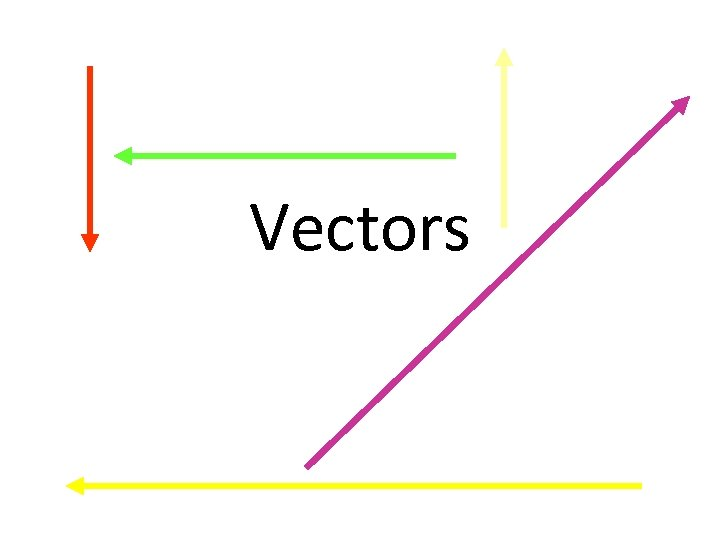Vectors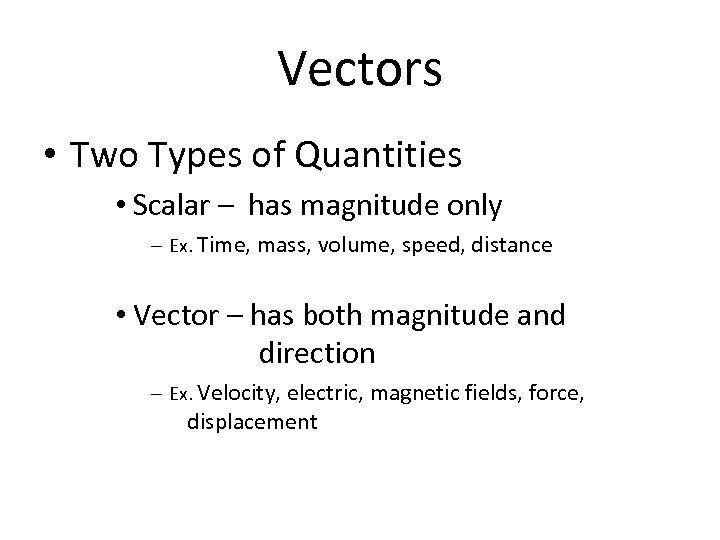Vectors • Two Types of Quantities • Scalar – has magnitude only – Ex. Time, mass, volume, speed, distance • Vector – has both magnitude and direction – Ex. Velocity, electric, magnetic fields, force, displacement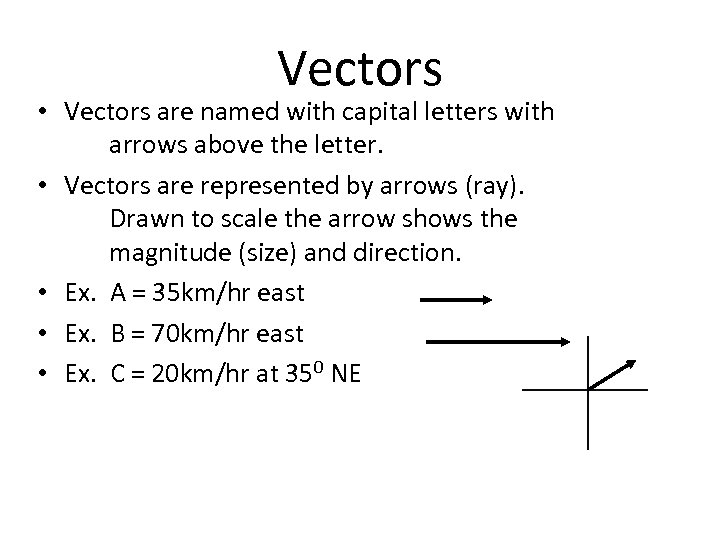Vectors • Vectors are named with capital letters with arrows above the letter. • Vectors are represented by arrows (ray). Drawn to scale the arrow shows the magnitude (size) and direction. • Ex. A = 35 km/hr east • Ex. B = 70 km/hr east • Ex. C = 20 km/hr at 350 NE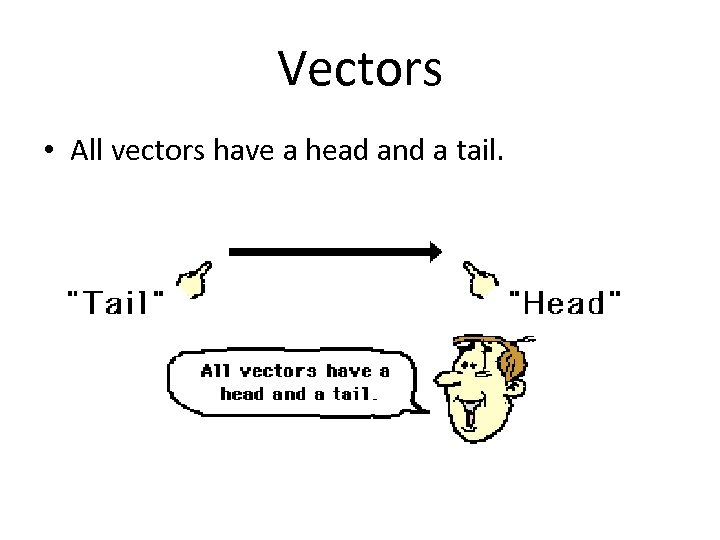Vectors • All vectors have a head and a tail.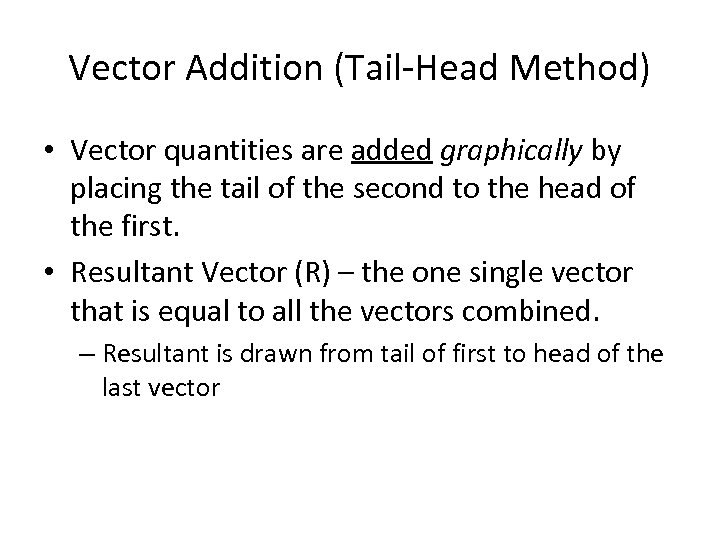Vector Addition (Tail-Head Method) • Vector quantities are added graphically by placing the tail of the second to the head of the first. • Resultant Vector (R) – the one single vector that is equal to all the vectors combined. – Resultant is drawn from tail of first to head of the last vector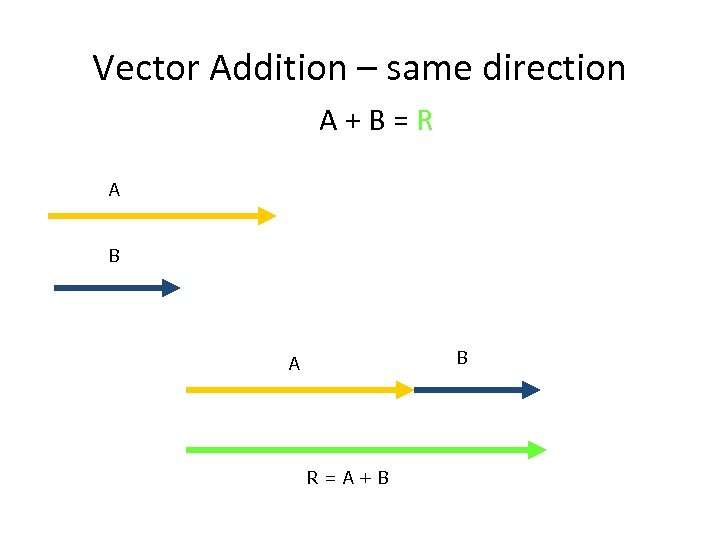Vector Addition – same direction A+B=R A B B A R=A+B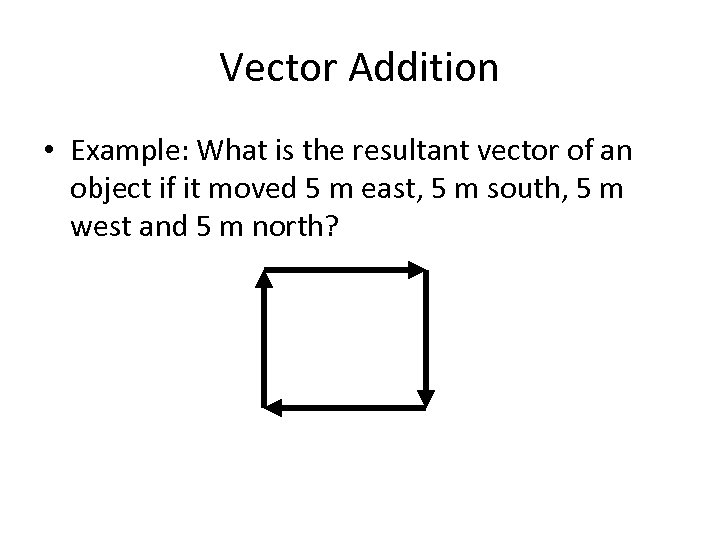Vector Addition • Example: What is the resultant vector of an object if it moved 5 m east, 5 m south, 5 m west and 5 m north?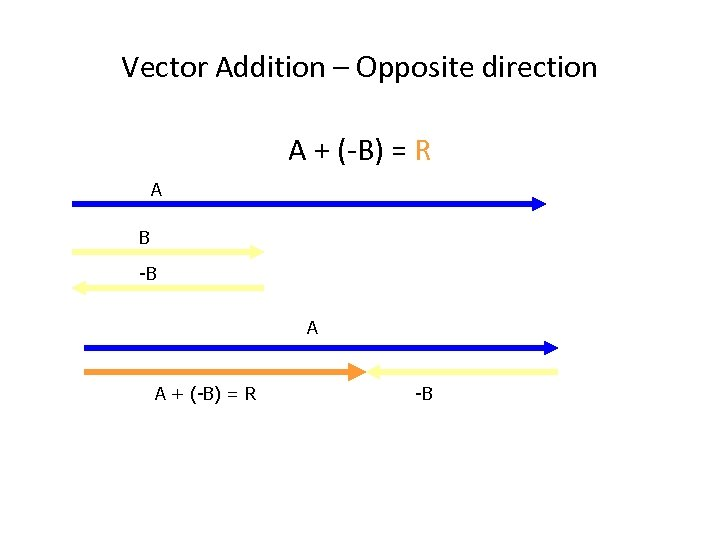Vector Addition – Opposite direction A + (-B) = R A B -B A A + (-B) = R -B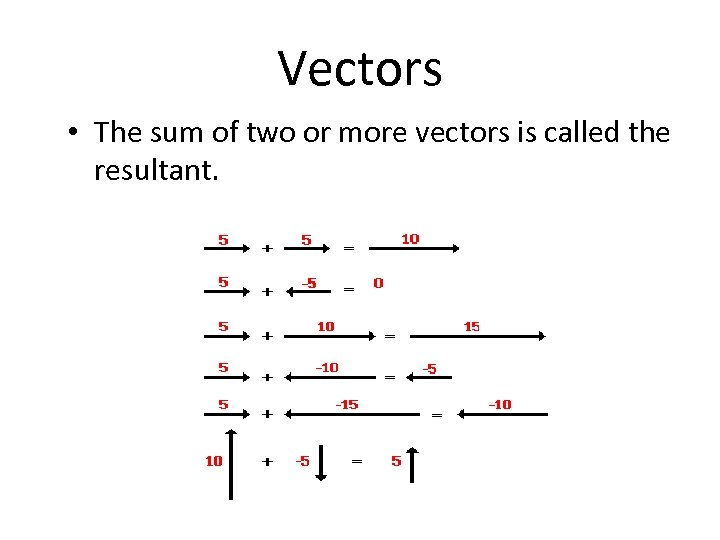Vectors • The sum of two or more vectors is called the resultant.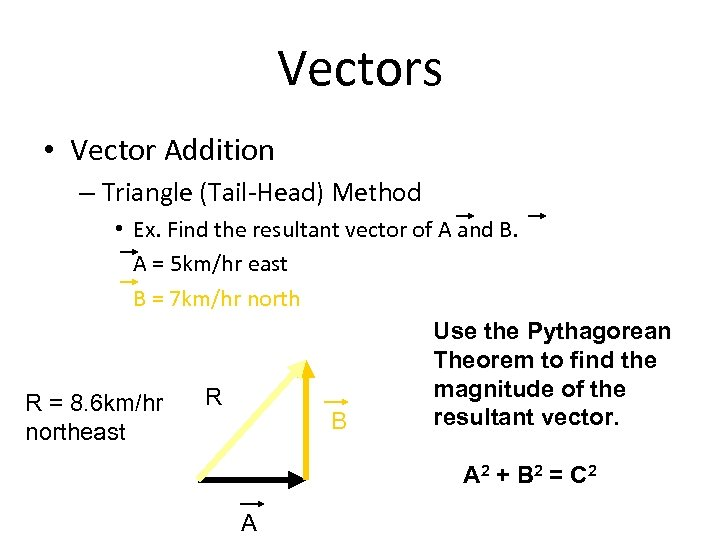Vectors • Vector Addition – Triangle (Tail-Head) Method • Ex. Find the resultant vector of A and B. A = 5 km/hr east B = 7 km/hr north Use the Pythagorean Theorem to find the magnitude of the R R = 8. 6 km/hr resultant vector. B northeast A 2 + B 2 = C 2 A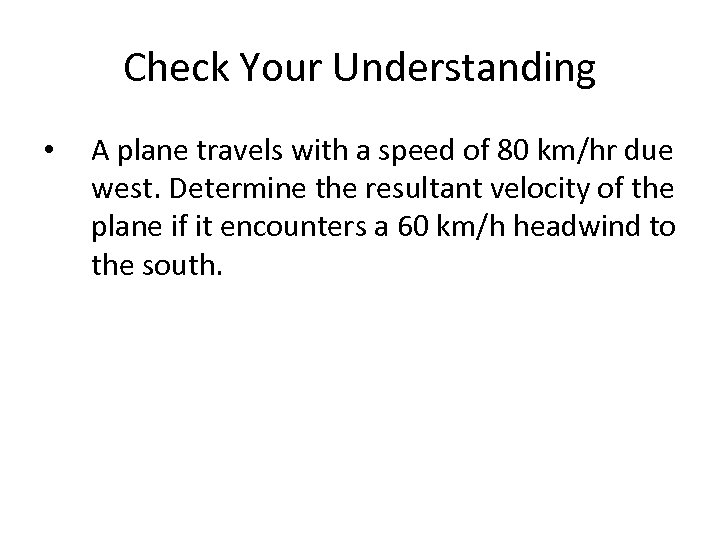Check Your Understanding • A plane travels with a speed of 80 km/hr due west. Determine the resultant velocity of the plane if it encounters a 60 km/h headwind to the south.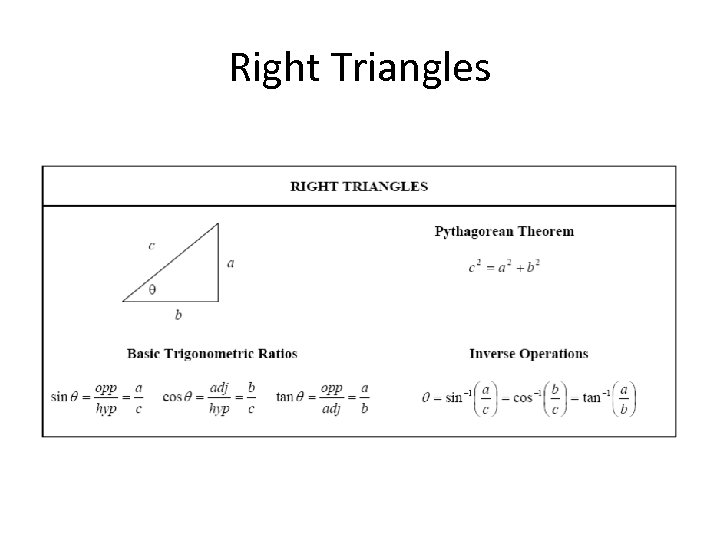Right Triangles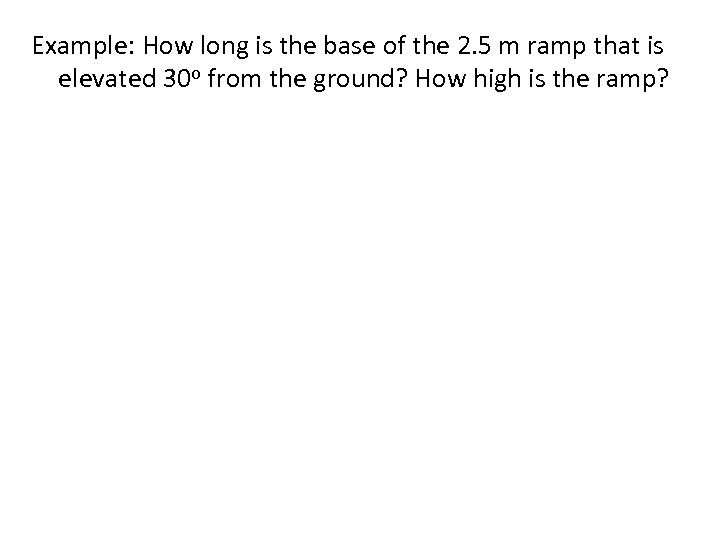Example: How long is the base of the 2. 5 m ramp that is elevated 30 o from the ground? How high is the ramp?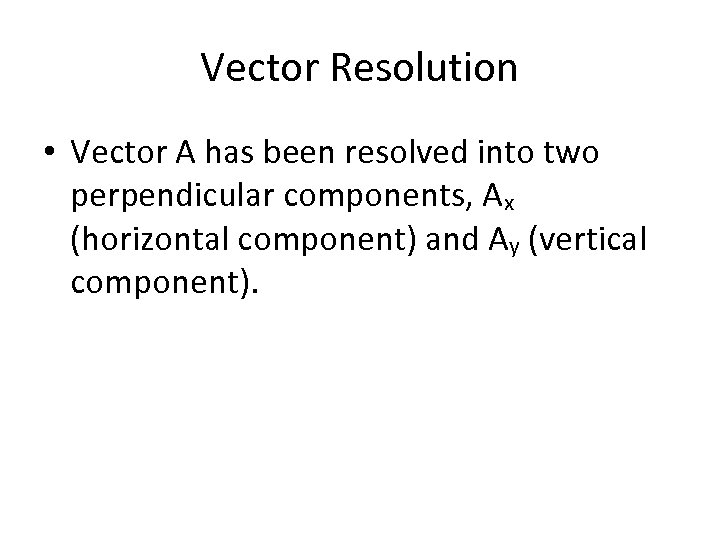Vector Resolution • Vector A has been resolved into two perpendicular components, Ax (horizontal component) and Ay (vertical component).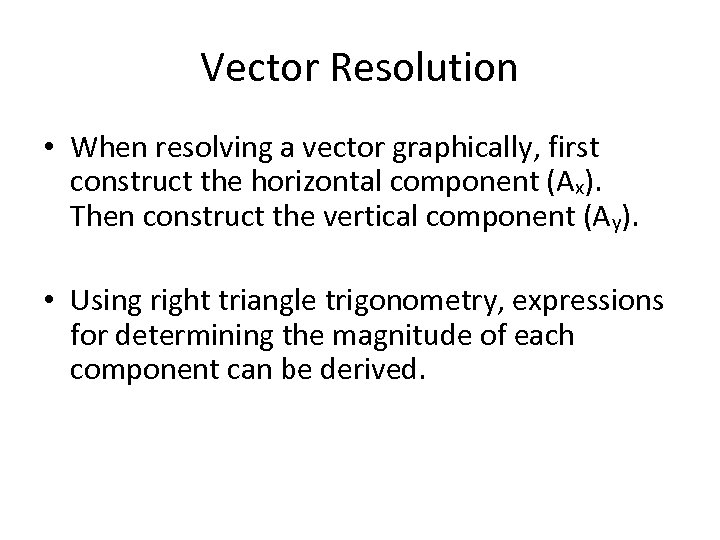Vector Resolution • When resolving a vector graphically, first construct the horizontal component (Ax). Then construct the vertical component (Ay). • Using right triangle trigonometry, expressions for determining the magnitude of each component can be derived.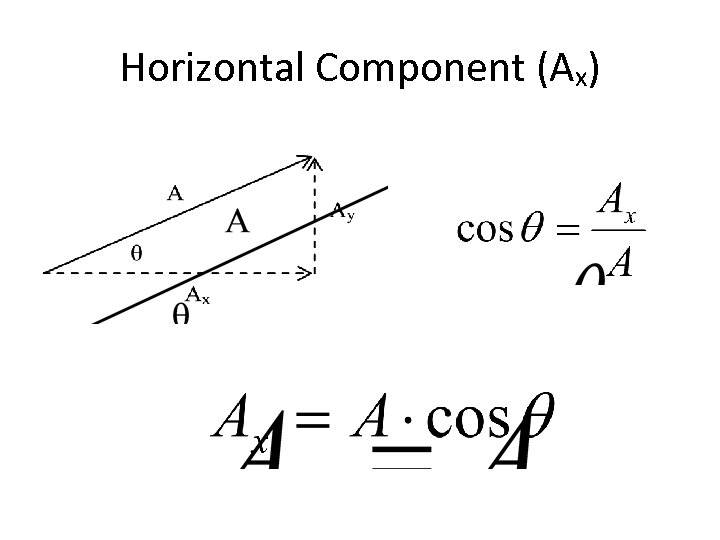Horizontal Component (Ax)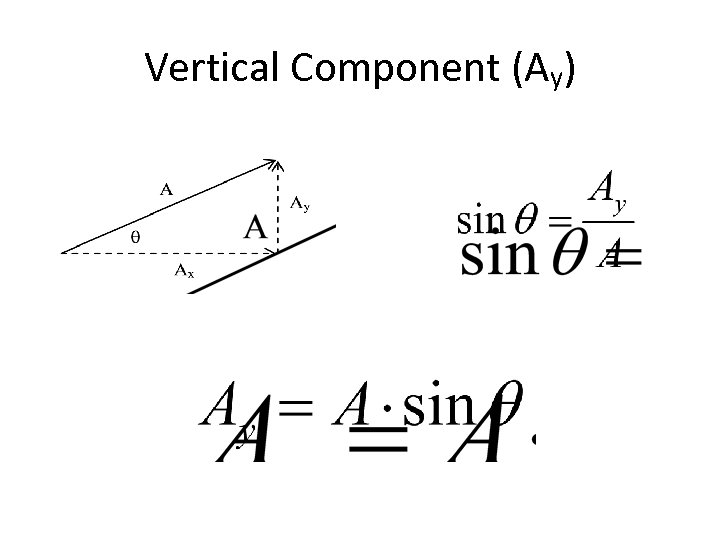Vertical Component (Ay)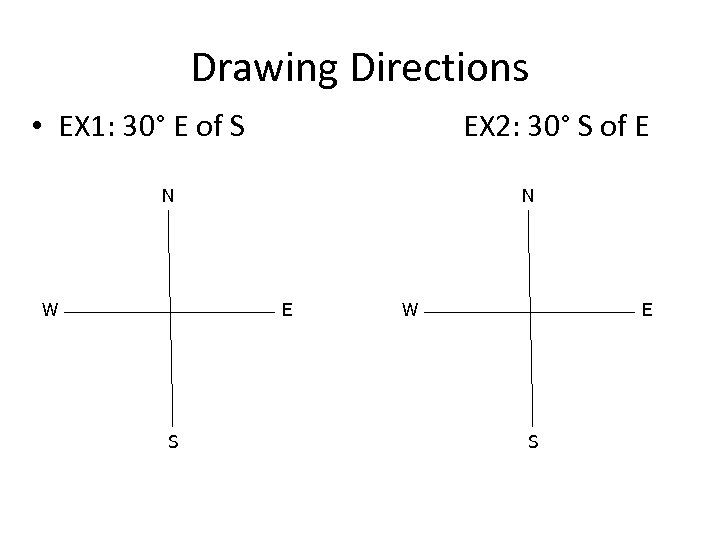Drawing Directions • EX 1: 30° E of S EX 2: 30° S of E N W N E S W E S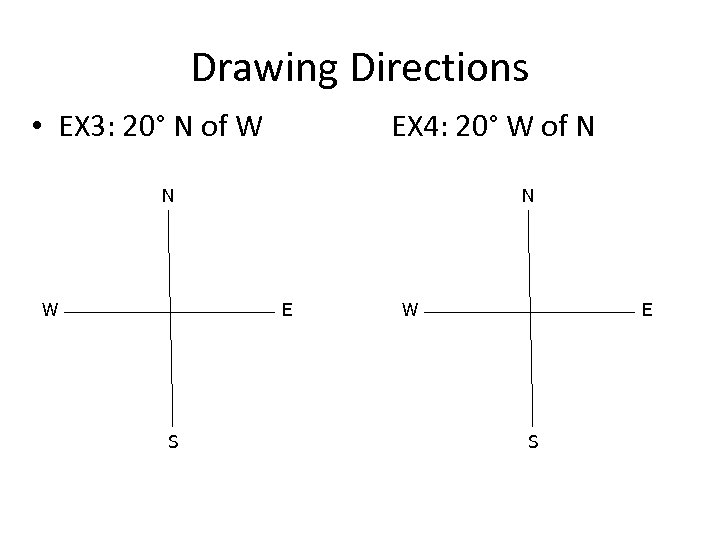Drawing Directions • EX 3: 20° N of W EX 4: 20° W of N N W N E S W E S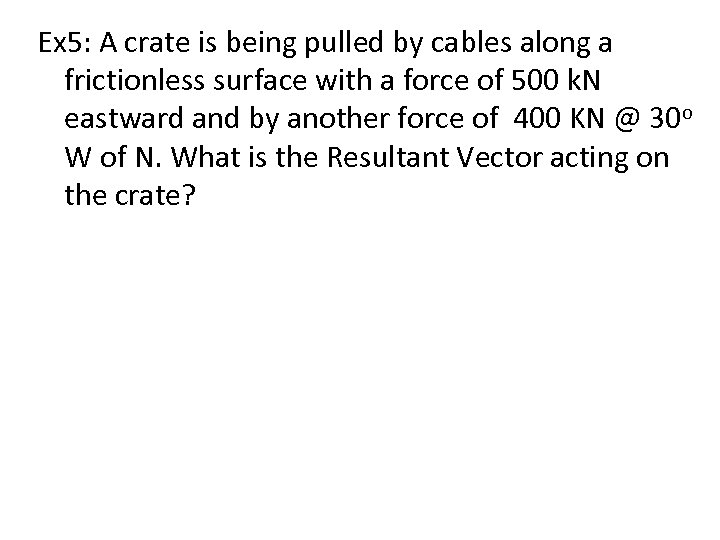Ex 5: A crate is being pulled by cables along a frictionless surface with a force of 500 k. N eastward and by another force of 400 KN @ 30 o W of N. What is the Resultant Vector acting on the crate?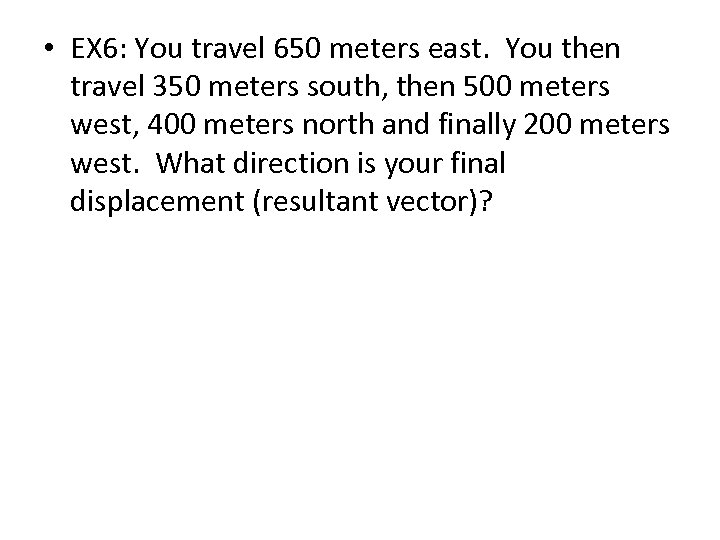• EX 6: You travel 650 meters east. You then travel 350 meters south, then 500 meters west, 400 meters north and finally 200 meters west. What direction is your final displacement (resultant vector)?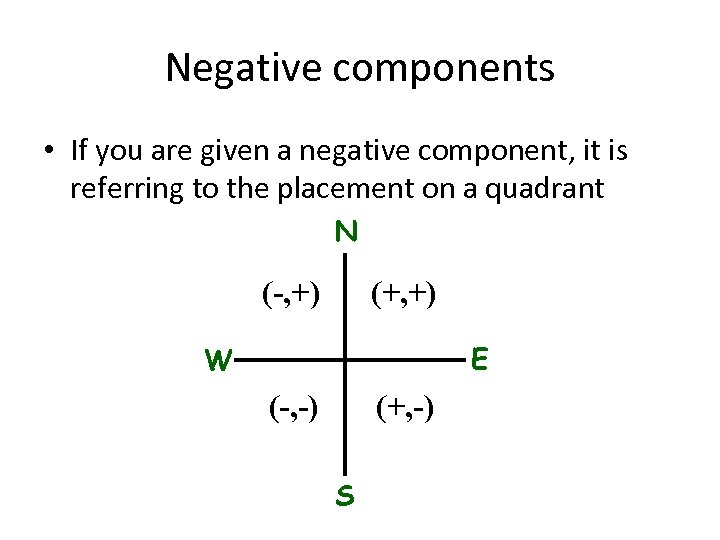Negative components • If you are given a negative component, it is referring to the placement on a quadrant N (-, +) (+, +) E W (-, -) (+, -) S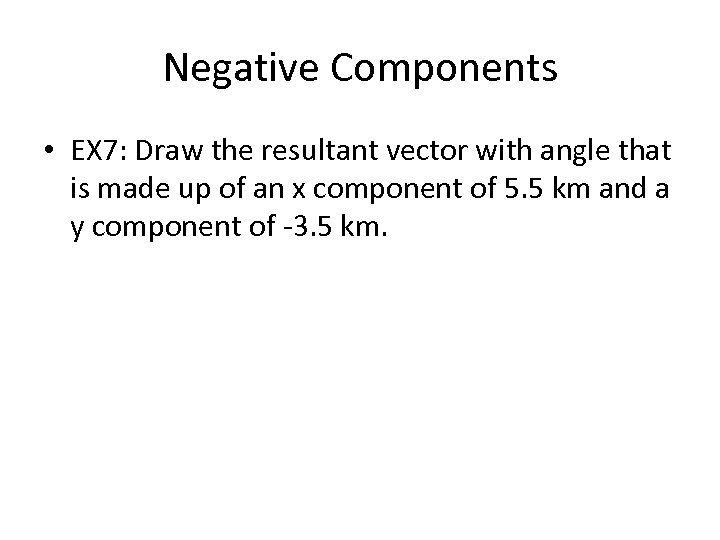Negative Components • EX 7: Draw the resultant vector with angle that is made up of an x component of 5. 5 km and a y component of -3. 5 km.